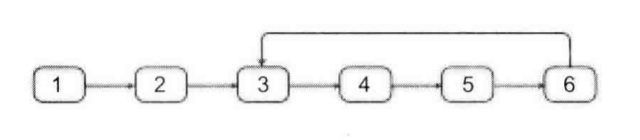# 链表中环的入口

### 样例[1, 2, 3, 4, 5, 6]
2


### 思路及证明过程

• 快慢指针法
graph LR f{FAST} -->|2| 1((HEAD)) -->|X| 2((Entrance)) -->|Y| 3((ptr)) -->|Z| 4((net)) --> |Y| 2((Entrance)) s{SLOW} -->|1| 1((HEAD))

HEADEntrance 之间的距离为 $X$ ，Entranceptr 之间的距离为 $Y$ ，当其相遇时，此时有 $L_=2(X + Y)$, $L_ = X + Y$

graph LR 1((HEAD)) -->|X| 2((Entrance)) -->|Y| 3((ptr)) -->|Z| 4((net)) --> |Y| 2((Entrance))

### Code

/**
* class ListNode {
*     int val;
*     ListNode next;
*     ListNode(int x) {
*         val = x;
*         next = null;
*     }
* }
*/
class Solution {
while (ptr != null && net != null) {
ptr = ptr.next; net = net.next;
if (net != null) {
net = net.next;
if (net == null) return null;
}
if (ptr == net) {